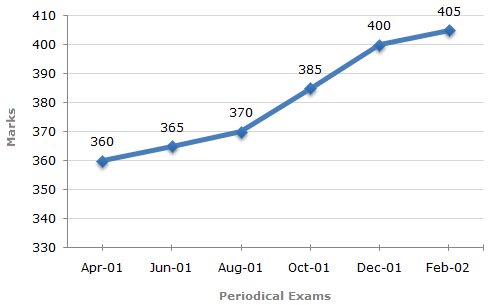# Data Interpretation - Line Charts - Discussion

### Discussion :: Line Charts - Line Chart 10 (Q.No.1)

In a school the periodical examination are held every second month. In a session during April 2001 - March 2002, a student of Class IX appeared for each of the periodical exams. The aggregate marks obtained by him in each perodical exam are represented in the line-graph given below.

Marks Obtained by student in Six Periodical Held in Every Two Months During the Year in the Session 2001 - 2002.

Maximum Total Marks in each Periodical Exam = 5001.

In which periodical exams did the student obtain the highest percentage increase in marks over the previous periodical exams ?

 [A]. June, 01 [B]. August, 01 [C]. Oct, 01 [D]. Dec, 01

Explanation:

Percentage increase in marks in various periodical exams compared to the previous exams are:

 For Jun 01 =(365 - 360) x 100% = 1.39%. 360

 For Aug 01 =(370 - 365) x 100% = 1.37%. 365

 For Oct 01 =(385 - 370) x 100% = 4.05%. 370

 For Dec 01 =(400 - 385) x 100% = 3.90%. 385

 For Feb 02 =(404 - 400) x 100% = 1.25%. 400

Clearly, highest percentage increase in marks is in Oct 01.

 Ramya said: (Aug 17, 2011) Is there any easy method to solve this?

 Sarvani said: (Aug 7, 2016) 100 * [(370 - 365)/365] = 4.1%. So, Ans: August.

 Shubhankar said: (Dec 29, 2019) Yes, there is one fast method. We can visibly calculate the difference between two value i.e. difference of (15) coming only in two different years. And when we divide the difference with the previous year value means the year which have larger value in denominator will give less value. Ex- 15/385, 15/370. Hope this will help.